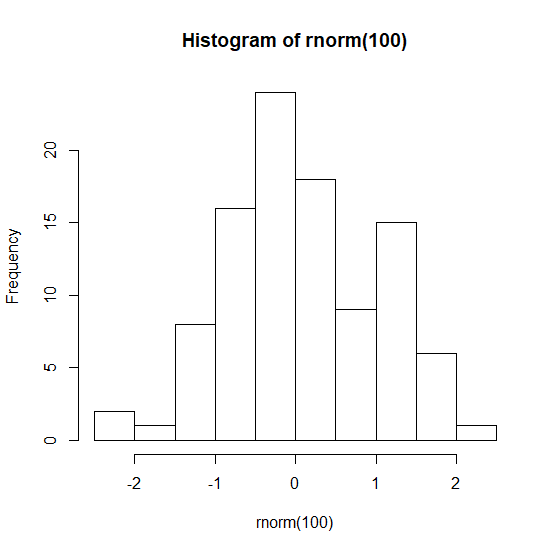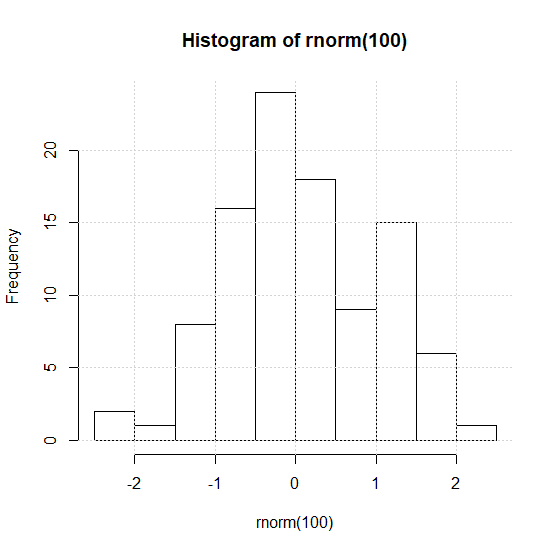# How to create a plot in R with gridlines using plot function?

Any plot created by using plot function does not display the plot with gridlines. On the other hand, if we create a plot using ggplot2 package then the plot has gridlines. Therefore, if we want to have gridlines on our plot then either we should create the plot using ggplot2 package or we can use the command grid() to add the gridlines on the plot created by plot function.

## Example

Creating a histogram using plot function −

> hist(rnorm(100))

## OutputAdding the grid lines to the above plot −

> grid()

## Output